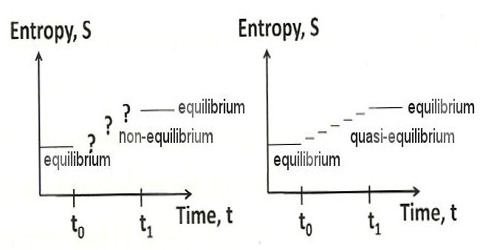# Entropy vs Equilibrium

Entropy vs equilibrium

A system is said to be in equilibrium when it has no further tendency of change. In a reversible process all stages are at equilibrium position. Since for a reversible process dS = 0, this must also be the condition of equilibrium. This implies that when the system is at equilibrium any change in the system will not lead to a change in the entropy of the system as long as the equilibrium condition is maintained. Conversely, if in a process the total change in entropy of the system turns out to be zero, the system is said to be in equilibrium.

The natural tendency of all systems is to approach a state of equilibrium. In mechanical systems the state of equilibrium is the state at which the system has minimum potential energy. The equilibrium conditions in a thermodynamic system may be expressed in terms of entropy. A spontaneous change in a system is accompanied by an increase in entropy. In equilibrium, the entropy of the system cannot increase (because it is already at a maximum) and it cannot decrease (because that would violate the second law of thermodynamics). In such a process, the entropy will increase until no further spontaneous change can occur. Under this condition the entropy of the system maximum and a state of equilibrium has been established. This may be described as thermodynamic equilibrium. A criterion of such equilibrium is;

In a system at equilibrium the entropy is maximum.